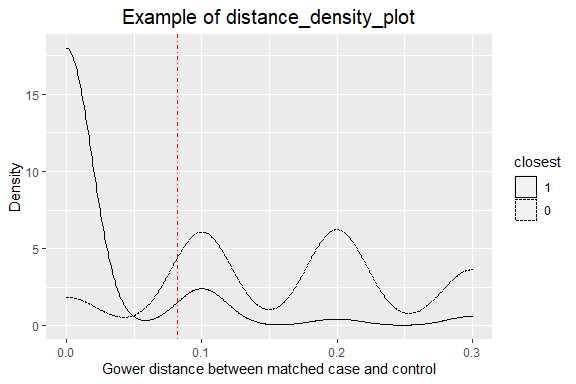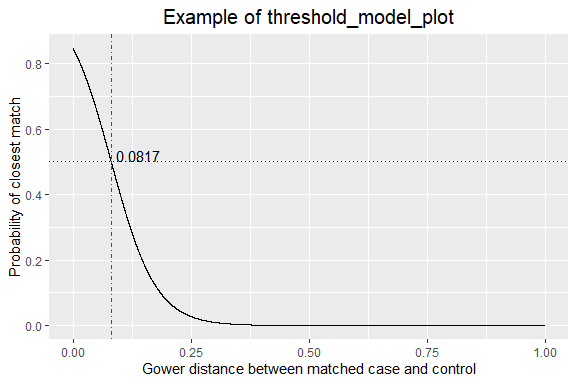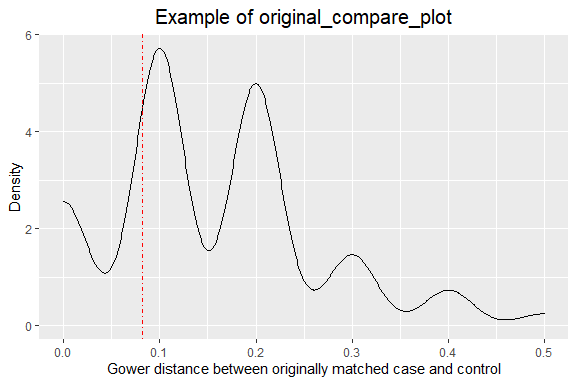# nncc: nearest-neighbors matching for case-control data

library(nncc)
library(survival)
library(dplyr)
library(ggplot2)
data(anifood)

## 1. Prepare the data

Case-control studies often collect many exposures but have relatively small sample sizes in comparison to the number of exposures. Conventional analysis techniques like multivariate logistic regression can only accommodate a limited number of exposures. The nncc package was designed to help address this limitation. nncc matches a case to its nearest available neighbors based on a user-defined measure of distance (by default Gower distance) calculated using all collected confounders for a given exposure. In the end, nncc creates a matched data set for each exposure of interest.

Ideally, one prefers to follow the analysis plan pre-defined at study design. Decisions to change your analysis plan to this approach should be considered carefully in light of how they may affect bias and uncertainty in model estimates. In addition, constructing variables are a routine and sometimes necessary part of multifactor studies. However, constructing new outcome variables from primary variables will increase dependencies and the difficulty of interpretation, especially where there is missingness of data.

For illustration, we created a toy example case-control data set called anifood which only contains 250 cases, 250 controls, and 11 variables including the one indicating case status, case, which equals 0 for controls, 1 for cases.

dim(anifood)
#>  500  11

In addition to the data set, nncc needs to know the exposures of interest, the variables used for matching, and a data set that contains variables to be excluded from matching for each exposures (e.g., possible intermediates in the pathway between an exposure and case status).

# exposures of interest. In a real study, this list can be much longer
exp_interest <- c("exp01","exp09", "exp27")

# exposures to be controlled for any exposure of interest
exp_match <- setdiff(names(anifood), "case")

# variables to be excluded from matching for each exposure
# both exp_var and rm_vars are character variables
#>   exp_var            rm_vars
#> 1   exp01             exp09
#> 2   exp09       exp24 exp27
#> 3   exp27 exp43 exp45 exp50

## 2. Establish a threshold for distance

The first step is to establish a proper distance threshold for matching. If too loose, there will be poor confounding control; if too tight, it will be difficult to find enough controls for the cases due to overmatching.

The get_threshold function uses the maximum bipartite graph algorithm to find each case’s closest control while ensuring each control is used no more than once. Then, for each case, a control is selected randomly.

A logistic regression on whether the control is the closest or the randomly selected one based on the distance is created. By default, the threshold used is the distance at which the probability is at least 50% that the control is the closest vs. a randomly selected one (see the plot below generated by the function threshold_model_plot). You can choose a different probability with the p_threshold argument.

threshold_results <- get_threshold(anifood, exp_match, p_threshold = 0.50)
#> case

The distance_density_plot function plots the density distribution of Gower distances for the maximum bipartite graph algorithm matches (solid line), the density distribution of distances for the random matches (dashed line), and the threshold (red dashed line). As expected, a maximum bipartite graph algorithm matching results in closer matching than a random matching.

distance_density_plot(threshold_results) + ggtitle("Example of distance_density_plot")The function threshold_model_plot plots the probability of being the maximum bipartite graph algorithm match vs. a random one by distance. It also plots the threshold for distance corresponding to a probability specified through the p_threshold argument.

threshold_model_plot(threshold_results, p_threshold = 0.50) + ggtitle("Example of threshold_model_plot")This package can also be used to analyze data from a case-control study that was originally matched. The original_compare_plot function makes a density plot of the distance for originally matched pairs. It also gives the proportion of originally matched pairs that have a distance greater than the threshold.

# create a variable (i.e., pair) indicating originally matched pairs
anifood_matched <- anifood %>% group_by(case) %>% mutate(pair = seq_along(case)) %>% ungroup

In this example, 84% of original matched case-control pairs have a distance greater than the threshold.

p <- original_compare_plot(anifood_matched, case, pair, threshold_results)

# the density plot of distance between originally matched cases and controls
p$plot_density + ggtitle("Example of original_compare_plot")# proportion of originally matched cases and controls with a distance greater than the threshold p$prop_distance_gt_threshold
#> .
#>     FALSE      TRUE
#> 0.1627907 0.8372093

## 3. Create strata: candidate matches for final analysis

The make_knn_strata function calculates Gower distances, by default, for pairs formed by every case and every control for a given exposure and creates strata by selecting the closest 250 controls for each case (by default, can be modified through the ncntls argument). Thus, for that exposure, the number of rows in data frame equals the number of cases * 251. The data frames for all exposures are stored in a list with a length equal to length(exp_interest).

This step can be computationally intensive depending on the amount of data. furrr::future_map() can be used so that the computation can be easily adjusted to use multiple cores on your local computer or a high-performance computing (HPC). More information is provided below.

library(furrr)
strata1 <- future_map(exp_interest, make_knn_strata,  rmvars = excl_vars, matchvars = exp_match, df = anifood) %>%
setNames(exp_interest)
length(strata1) == length(exp_interest)
#>  TRUE

# rows in a matched data set
all.equal(anifood %>% filter(case == 1) %>% NROW %>% *(250 + 1), NROW(strata1[]))
#>  TRUE

In the make_knn_strata function, a data frame should be supplied to the rmvars argument (see excl_vars above). That data frame should include two variables: exp_var: a character variable (only a single exposure in a row), and rm_vars: a character variable that contains the names of the variables that are not adjusted for. Names are separated by a space.

## 4. Prepare final matched sets for analysis

For a given exposure, the make_analysis_set function selects the closest 20 controls for each case; subset these 20 to those that fall within the threshold; collapse strata that share the same controls; and remove strata without any control.

strata2 <- future_map(exp_interest, make_analysis_set, stratified_data = strata1, data = anifood, maxdist = threshold_results$threshold) %>% setNames(exp_interest) #> exp01 #> exp09 #> exp27 The finalize_data function ensures that a control retained in a data frame is used once; removes strata without any case or any control. In this process, priority is given to the smallest strata then smallest distance if a control is matched to multiple cases (i.e., that control exists in multiple strata). strata3 <- finalize_data(strata2) The last step is to exclude exposures, if any, with odds ratios that are not estimable (e.g., none of cases and controls was exposed). For example, exp09 in this example. # exposures to which neither cases nor controls were exposed strata3 %>% lapply(function(dfm) dfm %>% mutate(case = as.character(case), exp = as.character(exp)) %>% filter(case != "" & exp != "") %>% with(table(case, exp))) %>% lapply(function(x) x==0) %>% lapply(function(x) {sum = sum(x); length = length(x); cbind(sum, length)}) %>% do.call(rbind,.) %>% as.data.frame %>% mutate(var = names(strata3)) %>% filter(sum >= 2 | length < 4) %>% select(var) %>% unclass %>% unlist -> expvars_invalid expvars_invalid #> character(0) # none exposed and odds ratio cannot be estimated strata3[["exp09"]] %>% with(table(case, exp)) #> exp #> case 0 1 #> 0 236 0 #> 1 231 1 data_final <- strata3[setdiff(names(strata3), expvars_invalid)] ## 5. Calculate odds ratios ### 5.1 Mantel–Haenszel test and Fisher’s exact test The test_mh function performs the Mantel–Haenszel test for data frame with more than one stratum and the Fisher’s exact test for a data frame with only one stratum. or_mh <- future_map(data_final, function(dfm) with(dfm, test_mh(case = case, exp = exp, strata = strata))) or_mh[["exp01"]] #>$p.value
#>  0.727695
#>
#> $conf.int #>  0.7440193 1.5998105 #> attr(,"conf.level") #>  0.95 #> #>$estimate
#> common odds ratio
#>          1.091004
#>
#> $null.value #> common odds ratio #> 1 #> #>$alternative
#>  "two.sided"
#>
#> $method #>  "Mantel-Haenszel chi-squared test with continuity correction" #> #>$data.name
#>  "case and exp and strata"

However, when the number of exposed is small, this method may yield an infinite result.

data_final[["exp27"]] %>% select(case, exp) %>% table
#>     exp
#> case   0   1
#>    0 236   0
#>    1 231   1

or_mh[["exp27"]]
#> $p.value #>  0.9698768 #> #>$conf.int
#>  NaN NaN
#> attr(,"conf.level")
#>  0.95
#>
#> $estimate #> common odds ratio #> Inf #> #>$null.value
#> common odds ratio
#>                 1
#>
#> $alternative #>  "two.sided" #> #>$method
#>  "Mantel-Haenszel chi-squared test with continuity correction"
#>
#> $data.name #>  "case and exp and strata" ### 5.2 Conditional logistic regression Conditional logistic regression gives results similar to those from Mantel–Haenszel test, but may also give less than ideal results when few participants are exposed. results_clogit <- future_map(data_final, function(dfm){clogit(case ~ exp + strata(strata) , data = dfm)}) results_clogit[["exp27"]] %>% summary() %>% $(conf.int)
#>      exp(coef)   exp(-coef) lower .95 upper .95
#> exp1  11766455 8.498736e-08         0       Inf

### 5.3 Penalized logistic regression

When the number of participants being exposed is small, Firth’s bias-Reduced penalized-likelihood logistic regression can be used (1).

# regression coefficients
coef_logistf <- future_map(data_final, function(dfm){

if(length(unique(dfm[["strata"]])) == 1) {
x <- model.matrix(case ~ exp, data = dfm)
plconf <- grep("exp", colnames(x))
o <- logistf(case ~ exp, data = dfm, plconf = plconf, dataout = FALSE) %>%
[(c("terms", "coefficients", "ci.lower", "ci.upper", "prob"))
} else {
x <- model.matrix(case ~ exp + strata, data = dfm)
plconf <- grep("exp", colnames(x))
o <- logistf(case ~ exp + strata, data = dfm, plconf = plconf, dataout = FALSE) %>%
[(c("terms", "coefficients", "ci.lower", "ci.upper", "prob"))
}
},
.progress = TRUE)

# odds ratios
or_logistf <- lapply(names(coef_logistf), function(var_name){
coef_logistf[[var_name]] %>%
[(c("terms", "coefficients", "ci.lower", "ci.upper", "prob")) %>%
bind_cols %>%
filter(grepl("exp", terms)) %>%
mutate(variable = var_name, or = exp(coefficients), ci.lower = exp(ci.lower), ci.upper = exp(ci.upper)) %>%
select(variable, terms, or, ci.lower, ci.upper, prob)}) %>%
setNames(names(coef_logistf))

# odds ratio for exp27
or_logistf[["exp27"]]
#> # A tibble: 1 x 6
#>   variable terms    or ci.lower ci.upper  prob
#>   <chr>    <chr> <dbl>    <dbl>    <dbl> <dbl>
#> 1 exp27    exp1   3.30    0.172     486. 0.434

## 6. Calculate population attributable fraction (PAF)

PAF can be estimated using the method described by Bruzzi and colleagues (2). We implemented this method in get_paf.


# prepare a data frame for calculating PAF
df_or <- bind_rows(or_logistf)

df_or
#> # A tibble: 3 x 6
#>   variable terms    or ci.lower ci.upper  prob
#>   <chr>    <chr> <dbl>    <dbl>    <dbl> <dbl>
#> 1 exp01    exp1   1.09    0.744     1.60 0.656
#> 2 exp09    exp1   3.54    0.182   523.   0.408
#> 3 exp27    exp1   3.30    0.172   486.   0.434
# point estimate of PAF
paf <- get_paf(df_or = df_or, which_or = or, exp_var = variable, exp_level = terms, df_matched = data_final)

paf
#> # A tibble: 3 x 2
#>    paf_or variable
#>     <dbl> <chr>
#> 1 0.0549  exp01
#> 2 0.00309 exp09
#> 3 0.00300 exp27

# lower confidence limit of PAF
paf_ci.lower <- get_paf(df_or = df_or, which_or = ci.lower, exp_var = variable, exp_level = terms, df_matched = data_final)

## 7. Multiple imputation of missingness

When the case-control data contains a large proportion of missingness, multiple imputation may be useful.

• For regression coefficients generated by Mantel–Haenszel test or conditional logistic regression, Rubin’s rule may be proper for combining the results (3).

• For Firth’s bias-Reduced penalized-likelihood logistic regression, the regression coefficients are not normally distributed. The results from imputed data sets should be combined using penalized likelihood profiles (4). Penalized likelihood profiles are implemented in logistf::CLIP.confint(), but the function was developed to combine results from imputed data sets that have the same structure. However, if we impute the original case-control data, and then apply nearest-neighbors matching to the imputed data, the final analytic data sets for a given exposure could have inconsistent structures because they could have different numbers of strata.

Thus, we modified the logistf::CLIP.confint() to accommodate our method. The modified function is included in the nncc package and is called CLIP.confint.difflevel(). Please cite the original paper (4) and/or the logistf package if you use this modified function for publication.

## 8. Helper function cacheit()

When the number of exposures of interest or the sample size of the original case-control study is large, it may be time-consuming for some analyses (e.g., strata creation). You may not want to have the same code evaluated again when you run your analysis later. The helper function cacheit() caches the results for you. Specifically, before your run cacheit(), create a folder named “cache” in the working directory of your project. When you run cacheit("abc", code) for the first time, it saves the results of your code as abc.rds in the cache folder; next time, when you run the code, it directly read the saved results in without evaluating your code.

strata1 <- cacheit("abc",
future_map(exp_interest, make_knn_strata,  rmvars = excl_vars, matchvars = exp_match, df = anifood) %>%
setNames(exp_interest),
clearcache = FALSE)

## 9. Run the analysis on your local computer or HPC

Considering the intensive computation for this approach, especially when multiple imputation is conducted, a future can be used to accelerate the analysis. Below we briefly introduce the use of futures on your local multi-core computer and HPC.

### 9.1 local computer

If you have a multi-core computer, the following code can assign a specified number of cores for the analysis.

library(nncc)
library(dplyr)
library(furrr)
library(future.batchtools)
# the workers argument is used to define the number of cores for the analysis. By default, all cores will be used.
plan(multisession, workers = 3)

strata1 <- future_map(exp_interest, make_knn_strata,  rmvars = excl_vars, matchvars = exp_match, df = anifood) %>%
setNames(exp_interest)

### 9.2 HPC

To run futures on HPC, you may need a template file, a job script, and a R script. The template file and the job script are created using a Linux application such as nano and saved as .sh files.

There are some exemplary template files here. The template file should be saved as batchtools.sge.tmpl in your current working directory or as .batchtools.sge.tmpl in your home directory on the HPC. Below is an example of a template file for Sun Grid Engine.

#!/bin/bash

## name of the job
#$-N <%= job.name %> ## tell the queue system to use the current directory as the working directory ## Or else the script may fail as it will execute in your top level home directory /home/username #$ -cwd

## Use environment variables
#$-V ## specify a queue #$ -q <%= resources$queue %> ## free to add other modules if necessary module load R/3.6.2 Rscript -e 'batchtools::doJobCollection("<%= uri %>")' exit 0 Below is an example of a job script to be submitted to the scheduler through a shell: #!/bin/bash -l # name of the job is helloR #$ -N helloR

#$-cwd #$ -V

#$-pe smp 2-12 #$ -q all.q

# to execute fugure-map.R
Rscript future-map.R

exit 0

The R code looks like:

library(furrr)
library(future.batchtools)

plan(batchtools_sge)

future_map(<a_list_or_vector>, function(x){...}, .progress = TRUE)